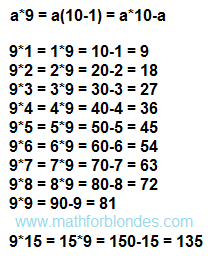## 4/20/2015

### 9 multiply 7 equals

Immediately write a ready answer: 9 multiply 7 equals 63. So we have the multiplication table says that we all have been taught, but to the end, many of us have not learned. I'm not an exception. Natural laziness and chronic multiple sclerosis did not allow me to remember this vital piece of mathematics. But it is knowledge of mathematics allows me to easily cope with this column multiplication tables. I just calculate the result I needed.

We all know that when multiplied by a permutation of the factors does not change the result. If somewhere sometime mathematics will tell you otherwise, do not believe them - they are wrong. Simple math multiplication like to call it, that in fact is not a multiplication. For example, if we call a cow "Multiplication", it then becomes to turn milk and poop in the hay. After all, the cow to the mathematical action has nothing to do.

So, I always use the following equation:

9*7 = 7*9

Next is quite simple. That number, which must be multiplied by 9, I multiply by 10 and take away his once:

7*9 = 7*10-7 = 70-7 = 63

Multiplied by 10 is very simple - have added to the number of the toe and the result is ready. Subtract this number from a small number of possible even without a calculator. Now you can easily cope with any stitch multiplication table 9.

For example, if we need to multiply 9 by 9, proceed as follows:

9*9 = 90-9 = 81

If needed multiply 9 by 3:

9*3 = 3*9 = 30-3 = 27

In all these transformations have no miracles, we just use the normal math.9 multiply

My personal opinion is: better to use the thinking abilities of their brain than clog the brain remembering any nonsense. Even if this nonsense forced to learn mathematics.